mixtures and alligation practice problems with solutions

mixtures and alligation practice problems with solutions
5 (100%) 2 vote[s]
Question 1: A grocer buys two kinds of rice at Rs. 1.80 and Rs. 1.20 per kg respectively. In what proportion should these be mixed, so that by selling the mixture at Rs. 1.75 per kg 25% may be gained?
[A] 2 : 1
[B] 3 : 2
[C] 3 : 4
[D] 1 : 2
Option # 4

Explanation

Solution :

S.P. Of 1 kg mixture =  Rs. 1.75,  gain= 25%.

Mean price= Rs.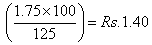(Dearer price) : (cheaper price)=20:40=1:2.

Question 2: A and B are two alloys of iron and silver prepared by mixing metals in the ratio 4 : 5 and 7 : 5 respectively. If equal quantities of alloys are melted to form a third alloy C, the ratio of iron to silver in C is
[A] 37 : 35
[B] 19 : 18
[C] 31 : 25
[D] 29 : 35
Option # 1

Explanation

Solution :

In alloy A, the ratio of iron to Silver=4:5, 5+4=9

In alloy B, the ratio of iron to Silver=7:5, 7+5=12, as the amount to be mixed.

So take the LCM of 9 and 12.  hence, we mix 36  gm of A and 36  gm of B.

(the reason for choosing LCM as the amount is to simplify calculations.)

For a: amount of iron=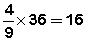And amount of silver=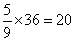For B: amount of iron=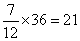And amount of silver=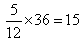Hence, Total amount of iron=16+21=37

Total amount of silver=20+15=35

Hence, Final ratio of iron to Silver in the mix of two alloys= 37:35

Shortcut: Required ratio is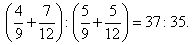Question 3: The ratio of milk and water in 66 kg of adultered milk is 5 : 1. Water is added to make the ratio 5 : 3. The quantity of water added is
[A] 22 kg
[B] 24.7 5kg
[C] 16.50 kg
[D] 20 kg
Option # 1

Explanation

Solution :

In the first mixture

Milk=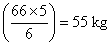Water=11kg

In the second mixture

If milk is 55kg, then water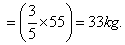Question 4: Mixture of alcohol and water contains 35% of alcohol by volume. Then, 40 ml of water is added to such a mixture of 100 ml. The percentage of alcohol in the new mixture is
[A] 30%
[B] 35%
[C] 25%
[D] 20%
Option # 3

Explanation

Solution :

Here percentage alcohol in the mixture is to be found out. So, the quantity of alcohol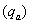is kept as numerator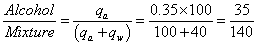(since 40 ml water is added

Quantity of mixture=100+40).

% alcohol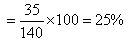the mixture contains 20% alcohol.

Question 5: A mixture, 729 ml in volume, contains milk and water in the ratio 7: 2. How much more water is to be added to get a new mixture containing milk and water in the ratio 7: 3?
[A] 600 ml
[B] 710 ml
[C] 520 ml
[D] None of   these
Option # 4

Explanation

Solution :

MilkWater=729-167=162 ml.

Let X ml of water be added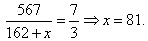Practie Problems Sets